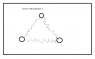# The entropy of a oscillator

• Tianwu Zang

## Homework Statement

In a reservoir there are three balls. There is a spring(the weight of spring is negligible) with elastic coefficient k between each two balls(small enough, like two particles). Suppose the center of gravity of the system does not move, and the mass of each ball is m. Suppose the temperature is T. The balance state is that the three balls are on the three vertices of an equilateral triangle with a side length L.

2. Questions
What is the entropy of this system?(This system is classical, non quantum) Consider this system as an ensemble.
And what is the normal mode vibration frequency of this system?

## The Attempt at a Solution

If it is an isolated problem, I can solve it. The Lagrangian is 1/2*m($$\dot{x1}$$2+$$\dot{y1}$$2+$$\dot{x2}$$2+$$\dot{y2}$$2+$$\dot{x3}$$2+$$\dot{y3}$$2)-1/2*k((x1-x2)2+(x1-x3)2+(x2-x3)2+(y1-y2)2+(y2-y3)2+(y1-y3)2), so the normal mode frequency can be solved by Lagrangian equation. But in this problem the system is no longer isolated. I am confused...
Thank you!

#### Attachments

•Untitled.png
2.8 KB · Views: 423
Last edited:
you could slightly pertrube the hamiltonian by infinitesimally moving the charges around the equilibrium point to get $$\delta H$$; this may lead you to entropy since from the problem there seems to be no work done on the system.

So my guess is $$dS = \frac{ \delta H}{T}$$

and i'd find more common degrees of freedom